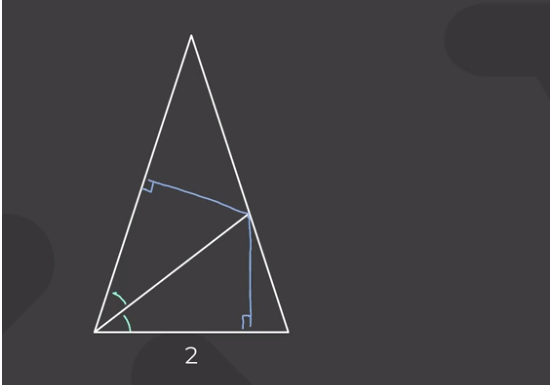# How can the hypotenuse alone determine the scaling factor here?

• Module 2 Week 3 Day 13 Challenge Part 1 Explanation

How can the hypotenuse alone determine the scaling factor here?

• @the-blade-dancer Hi there! This is an interesting question. There is a long train of logic that precedes this fact.Why is the ratio of the triangle areas based entirely on the ratio of the triangles' bases?

$$\hspace{ 30 mm}$$ ↑

It's because their heights are the same. ← Why is this true?

$$\hspace{ 70 mm}$$ ↑

$$\hspace{ 40 mm}$$ It's because the two right triangles are congruent.

$$\hspace{70 mm}$$ ↓ (see image)$$\hspace{40 mm}$$ ↑
$$\hspace{20 mm}$$ Why are the two triangles congruent?

$$\hspace{40 mm}$$ ↑
$$\hspace{20 mm}$$ It's because they each have a right angle and the $$\textcolor{green}{\text{green}}$$ angle.

$$\hspace{40 mm}$$ ↑

$$\hspace{20 mm}$$ Why do they both have a $$\textcolor{green}{\text{green}}$$ angle?

$$\hspace{40 mm}$$ ↑

$$\hspace{20 mm}$$ It's because of Angle Bisector Theorem!This is how Prof. Loh gets a ratio of $$\frac{(1+\sqrt{5})^2}{2^2}$$ for the area of the larger inner triangle to the area of the smaller inner triangle.• po shn loh crazy hand 2.0